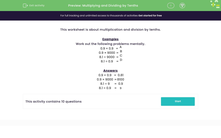# Practise Multiplying and Dividing by Tenths

In this worksheet, students will practise multiplying and dividing by tenths. They should first attempt their answers mentally.Key stage:  KS 3

Curriculum topic:   Number

Curriculum subtopic:   Use Four Operations for All Numbers

Difficulty level:#### Worksheet Overview

This activity is about multiplication and division by tenths.

Work out the following problems mentally.

Example 1

0.9 × 0.9

9 × 9 = 81

As we've multiplied both 0.9's by 10 we've multiplied by a total of 100. This means we will need to divide our answer by 100.

So, 0.9 × 0.9 = 0.81

Example 2

0.9 × 9,000

9 × 9,000 = 81,000

As we've multiplied 0.9 by 10 to get 9 we will need to divide our answer by 10.

So, 0.9 × 9,000 = 8,100

One of the easiest ways to multiply using decimals is to remove the decimal point, do the multiplication and then put the decimal point back, making sure that you have the same number of decimal places in the answer as in the original calculation.

Example 3

8.1 ÷ 9

81 ÷ 9 = 9

As we've multiplied 8.1 by 10 to get 81 we will need to divide our answer by 10.

So, 8.1 ÷ 9 = 0.9

Example 4

8.1 ÷ 0.9

81 ÷ 9 = 9

As we've changed the two values using the same proportion, we don't need to do anything to the answer we get.

Let's have a go at some questions now.

### What is EdPlace?

We're your National Curriculum aligned online education content provider helping each child succeed in English, maths and science from year 1 to GCSE. With an EdPlace account you’ll be able to track and measure progress, helping each child achieve their best. We build confidence and attainment by personalising each child’s learning at a level that suits them.

Get started